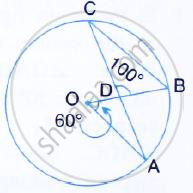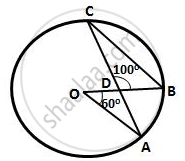Share

In the Following Figure, O is the Centre of the Circle, ∠Aob = 60° and ∠Bdc = 100° Find ∠Obc. - ICSE Class 10 - Mathematics

ConceptArc and Chord Properties - the Angle that an Arc of a Circle Subtends at the Center is Double that Which It Subtends at Any Point on the Remaining Part of the Circle

Question

In the following figure, O is the centre of the circle,
∠AOB = 60° and ∠BDC = 100° Find ∠OBC.SolutionHere, ∠ACB =1/2 ∠AOB=1/2 xx 60° = 30°
(Angle at the centre is double the angle at the circumference subtended by the same chord)
By angle sum property of ΔBDC,
∴ ∠DBC =180° - 100°- 30° = 50°
Hence, ∠OBC =50°

Is there an error in this question or solution?

Video TutorialsVIEW ALL 

Solution In the Following Figure, O is the Centre of the Circle, ∠Aob = 60° and ∠Bdc = 100° Find ∠Obc. Concept: Arc and Chord Properties - the Angle that an Arc of a Circle Subtends at the Center is Double that Which It Subtends at Any Point on the Remaining Part of the Circle.
S